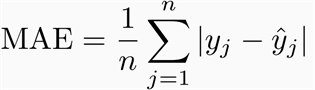• Angela Yu

# Machine Learning Basics - Model Evaluation 02

Updated: Feb 7, 2020

In the previous section, we discussed briefly the technique of train/test split and cross validation. In this section, we will investigate the mathematical intuition behind such procedure, as well as the metrics used for evaluating model performances both in terms of prediction accuracy and model efficiency.

## Topics:

- Bias/Variance Tradeoff

- Metrics

Accuracy: MSE/MAE/RMSE/R-squared

Efficiency: Adjusted R-squared/AIC/BIC/cpSuppose we are given a task: estimate y given a set of predictors X. We can prove thatThe second part of the equation represent the variance associated with the error term. It is irreducible and results from random sampling or factors outside our scope of investigation. The smaller the value of E(y-y-hat)squared, the smaller the error and better our prediction. Thus, our task appears to be a simple optimization problem. However, is it that simple?

We can also show that E(y-y-hat)squared can be decomposed into the sum of three fundamental quantities: the variance of y-hat, the squared bias of y-hat and the variance of the error term. Variance refers to the amount that our predicted value would change if we estimated our equation using a different training dataset; bias refers to the error that is introduced by approximating a real-life problem, which might be extremely complicated, by a much more simple model (ISLR, 35).Our optimization problem is thus accomplished if we could simultaneously achieve low variance and low bias. However, generally a more flexible model result in less bias but high variance (consider a model that works really hard to explain the unexplainable errors in this particular training set; the same error will not be present in a different dataset thus the parameter might be completely different), and a less flexible model result in more bias but high variance (we can imagine the extreme: predicting every y to be equal to 0, does that change with different dataset? Are we considering the complexity of the model we face?)

What is a flexible/complex model? A complex model constitutes the mathematical description of a complex object, the one that consists of interrelated component elements, that can also be constituted by their own interrelated elements.Thus, the total error reaches the minimum when we minimize both bias and variance simultaneously. However. given only one dataset to work with, we might be prone to develop a very complex model that works well with our training data (landing somewhere on the right of the above plot). However, if we use the complex model on other situations, we might find very high error rate due to high variance of our model. This the mathematical intuition behind the train-test split: we want to create an artificial representation of the real life problem to test our model's competence.From the previous variance/bias tradeoff image, we can observe the y axis is labelled: Total Error but how exactly do we calculate the total error, ie, how do we determine the performance of our model?

## - Metric One: Mean Square Error (MSE) and Root Mean Square Error (RMSE)MSE sums over all the data points, of the square of the difference between the predicted and actual target variables, divided by the number of data points. RMSE is the root square of the MSE. MSE measures how far off your predictions are from the actual values.

## - Metric Two: Mean Absolute Error (MAE)Mean absolute error measure the average magnitude of the errors in a set of predictions, without considering their direction.

## Difference between MAE, MSE and RMSE:

RMSE takes the square root of the average squared error, thus it gives relatively high weight to large error. If being off by 10 has more than twice the bad consequence as being off by 5, we choose RMSE because it gives relatively high weight to larger errors. The benefit of MAE is definitely in its interpretability.

## - Metric Three: R-squared and Adjusted R-squaredRsquared measures how much variation in our response variable is captured by our model. Adjusted Rsquare takes into consideration the number of predictors we use and will penalize the models with too many predictors.

## - Metric Three: CP/AIC/BIC

We will give more detailed explanation of the above when we discuss feature reduction methods.

## LAB:

13 views0 comments

See All